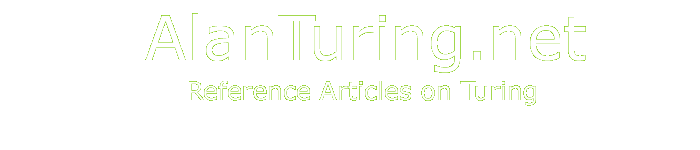# Turing's O-Machines

## By Jack Copeland

#### © Copyright B.J. Copeland, May 2000

O-machines are a type of abstract machine. They generate digital output from digital input by means of a step-by-step procedure consisting of repeated applications of a small, fixed number of atomic operations. The procedure unfolds under the control of a finite program of instructions which (as with the universal Turing machine) is stored in the form of data on the machine's tape.

Turing introduced the concept of an O-machine in his PhD thesis (Princeton, 1938). (The thesis was supervised by Alonzo Church.) This was subsequently published as 'Systems of Logic Based on Ordinals', Proceedings of the London Mathematical Society, series 2, vol. 45 (1939): 161-228. This paper is now a classic of recursive function theory.

The atomic operations of a Turing machine are six in number: (i) move the tape left one square; (ii) move the tape right one square; (iii) read (i.e. identify) the symbol currently under the head; (iv) write a symbol on the square of tape currently under the head (after first deleting the symbol already written there, if any); (v) change state; (vi) halt. These primitive operations are made available by unspecified subdevices of the machine - 'black boxes'. An O-machine is a Turing machine augmented with one or more atomic operations each of which returns the values of some function (on the natural numbers) that is not Turing-machine-computable. Each additional atomic operation is made available by a black box. Turing refers to these black boxes as 'oracles'. He remarks that an oracle works by 'unspecified means' and says that we need 'not go any further into the nature of [these] oracle[s]'.

Each oracle returns the values of a two-valued function. Let these values be written 0 and 1. Let p be one of the additional primitive operations. p is called into action by means of a special state c, the call state. (Where an O-machine has several of these additional primitives, a corresponding number of call states is required.) The machine inscribes the symbols that are to form the input to p on any convenient block of squares of its tape, using occurrences of a special symbol µ, the marker symbol, to indicate the beginning and the end of the input string. As soon as an instruction in the machine's program puts the machine into state c, the input is delivered to the subdevice that effects p, which then returns the corresponding value of the function.

The subdevice that effects p might simply write this value on the tape. On Turing's own way of handling matters, the value is not written on the tape; rather a pair of states, the 1-state and the 0-state, is employed in order to record values of the function. A call to p ends with a subdevice placing the machine in one or other of these two states according to whether the value of the function is 1 or 0.

When a function g is computable by an O-machine whose (only) oracle serves to return the values of a function f, then g is said to be computable relative to f.

One particular O-machine, the halting function machine, has as its 'classical' part the universal Turing machine specified by Turing in his paper of 1936 ( 'On Computable Numbers, with an Application to the Entscheidungsproblem') and as its 'nonclassical' part a primitive operation that returns the values of the halting function H(x,y).

The halting function is defined like this:

• H(x,y)=1 if and only if the xth Turing machine eventually halts if set in motion with the integer y inscribed on its tape, say in binary code (think of y as being the machine's input)
• H(x,y)=0 otherwise.

The halting function machine can compute many functions that are not Turing-machine-computable. This is because of the familiar phenomenon of the reducibility of one function to others (in the sense that multiplication is reducible to addition, for example). All functions reducible to the halting function and/or the atomic operations of an ordinary Turing machine are computable by the halting function machine.